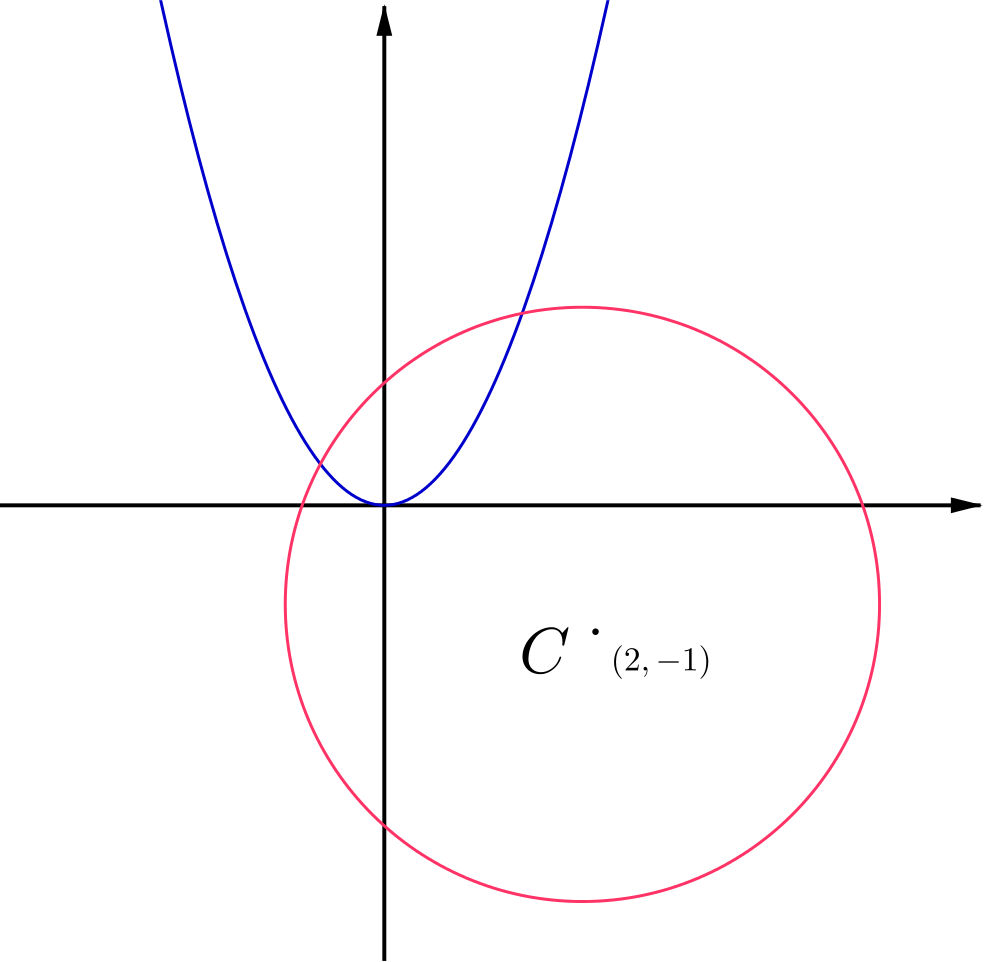Review question

# Can we show this quartic equation has exactly two real roots? Add to your resource collection Remove from your resource collection Add notes to this resource View your notes for this resource

Ref: R7549

## Solution

Sketch in the same diagram the graphs of $y = x^2$ and $x^2 + y^2 - 4x + 2y - 4 = 0$.

The graph of the equation $y = x^2$ is a parabola, while the graph of the equation $x^2 + y^2 - 4x + 2y - 4 = 0$ is a circle, since \begin{align*} x^2 + y^2 - 4x + 2y - 4 = 0 &\iff \left( (x-2)^2 - 4 \right) + \left( (y+1)^2 - 1 \right) - 4 = 0 \\ &\iff (x-2)^2 + (y+1)^2 = 9. \end{align*}

That is, the centre of the circle is $(2,-1)$ and the radius is $3$.Sketch of the parabola $y = x^2$ and the circle $x^2 + y^2 - 4x + 2y - 4 = 0$.

Hence or otherwise show that the equation $x^4 + 3x^2 - 4x - 4 = 0$ has two and only two real roots.

Consider the simultaneous equations \begin{align*} y &= x^2, \\ x^2 + y^2 - 4x + 2y - 4 &= 0. \end{align*}

As the diagram above shows, there are exactly two real solutions to this pair of equations.

If we substitute the first equation into the second, the second equation becomes $\begin{equation*} x^2 + (x^2)^2 - 4x + 2(x^2) - 4 = 0. \end{equation*}$ That is, $\begin{equation*} x^4 + 3x^2 - 4x - 4 = 0. \end{equation*}$

Thus $x^4 + 3x^2 - 4x - 4 = 0$ has exactly two real roots.

Find the positive root of the equation correct to one decimal place.

From our diagram, this root appears to be around $x = 1.4$.

With this in mind, we’ll let $\begin{equation*} f(x) = x^4 + 3x^2 - 4x - 4 \end{equation*}$

and calculate $f(x)$ for various values of $x$ around $x = 1.4$. By using a calculator, we have the following table.

$x$ $f(x)$
$1.3$ $-1.2739$
$1.4$ $0.1216$
$1.5$ $1.8125$

As there is a sign change and the function is continuous, the root we seek must fall between $1.3$ and $1.4$.

By using a calculator again, we see that $f(1.35) = -0.610994$, and so the root therefore falls between $1.35$ and $1.4$.

Thus, to one decimal place, the root is equal to $1.4$.

In fact, the root equals $1.392030791\!\ldots$.

We could also have located the root, to one decimal place, by using trial and improvement.